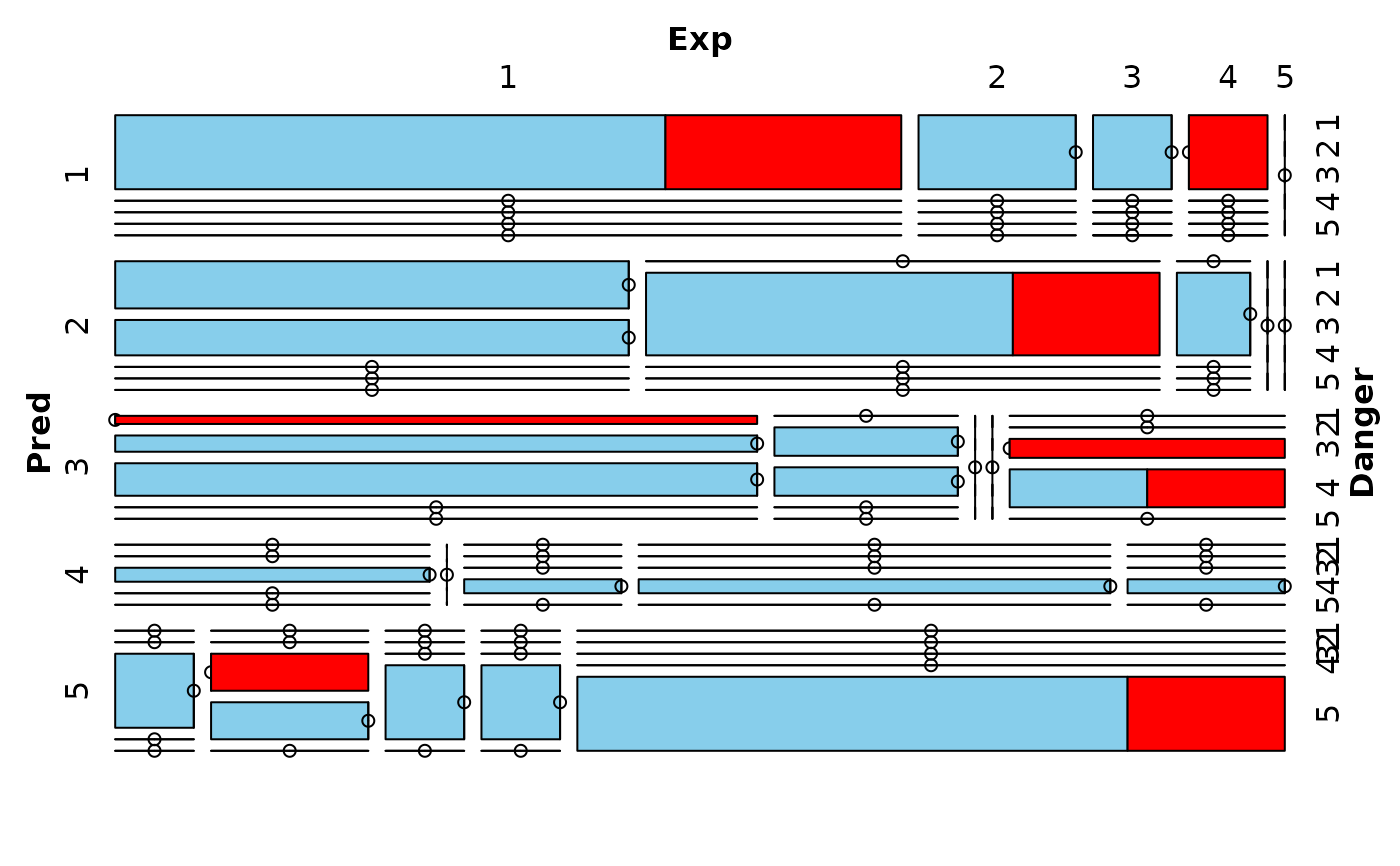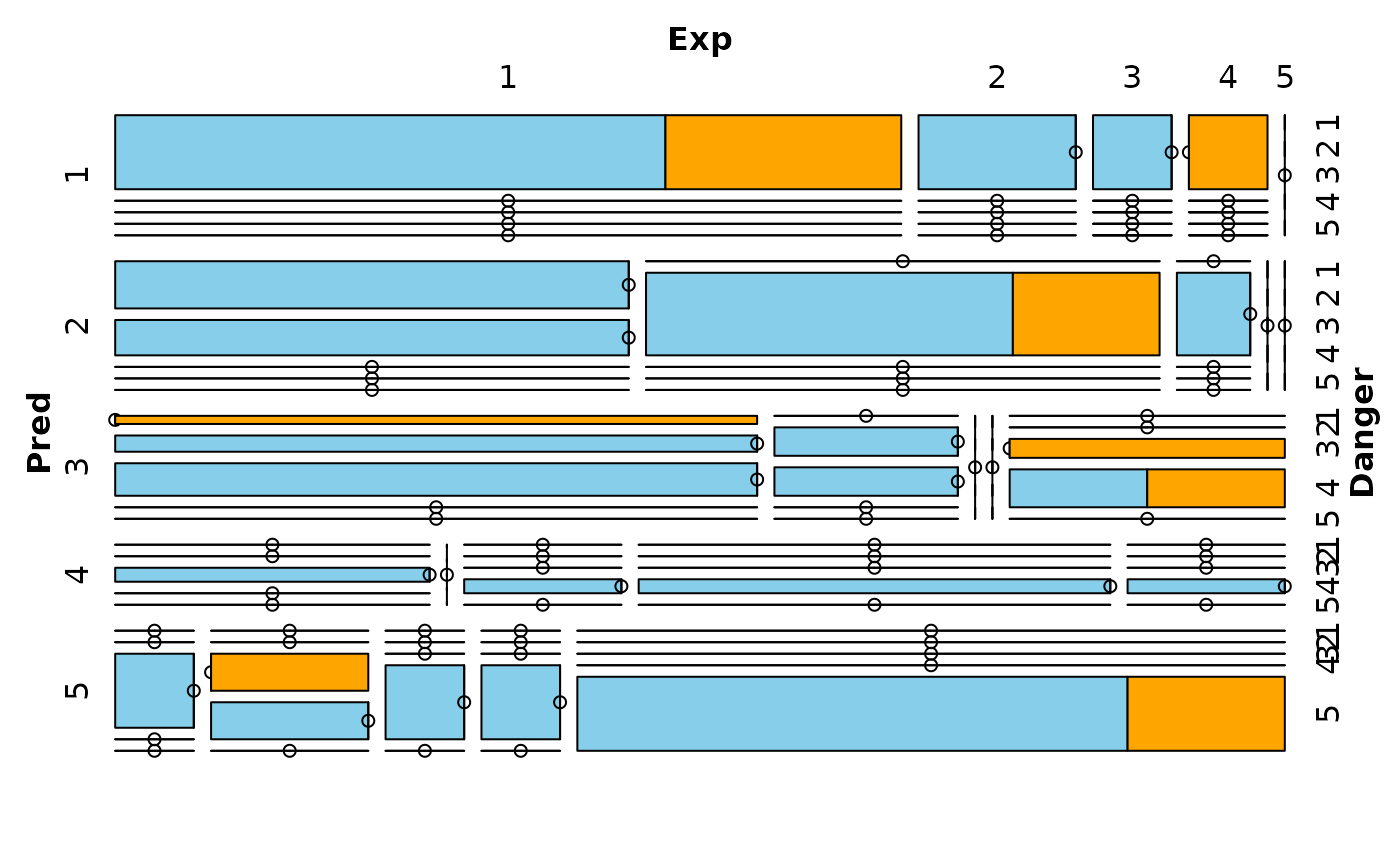Create a mosaic plot with information about missing/imputed values.

mosaicMiss(
x,
delimiter = NULL,
highlight = NULL,
selection = c("any", "all"),
plotvars = NULL,
col = c("skyblue", "red", "orange"),
labels = NULL,
miss.labels = TRUE,
...
)

## Arguments

x

a matrix or data.frame.

delimiter

a character-vector to distinguish between variables and imputation-indices for imputed variables (therefore, x needs to have colnames()). If given, it is used to determine the corresponding imputation-index for any imputed variable (a logical-vector indicating which values of the variable have been imputed). If such imputation-indices are found, they are used for highlighting and the colors are adjusted according to the given colors for imputed variables (see col).

highlight

a vector giving the variables to be used for highlighting. If NULL (the default), all variables are used for highlighting.

selection

the selection method for highlighting missing/imputed values in multiple highlight variables. Possible values are "any" (highlighting of missing/imputed values in any of the highlight variables) and "all" (highlighting of missing/imputed values in all of the highlight variables).

plotvars

a vector giving the categorical variables to be plotted. If NULL (the default), all variables are plotted.

col

a vector of length three giving the colors to be used for observed, missing and imputed data. If only one color is supplied, the tiles corresponding to observed data are transparent and the supplied color is used for highlighting.

labels

a list of arguments for the labeling function vcd::labeling_border().

miss.labels

either a logical indicating whether labels should be plotted for observed and missing/imputed (highlighted) data, or a character vector giving the labels.

...

additional arguments to be passed to vcd::mosaic().

## Value

An object of class "structable" is returned invisibly.

## Details

Mosaic plots are graphical representations of multi-way contingency tables. The frequencies of the different cells are visualized by area-proportional rectangles (tiles). Additional tiles are be used to display the frequencies of missing/imputed values. Furthermore, missing/imputed values in a certain variable or combination of variables can be highlighted in order to explore their structure.

## Note

This function uses the highly flexible strucplot framework of package vcd.

spineMiss(), vcd::mosaic()

Other plotting functions: aggr(), barMiss(), histMiss(), marginmatrix(), marginplot(), matrixplot(), pairsVIM(), parcoordMiss(), pbox(), scattJitt(), scattMiss(), scattmatrixMiss(), spineMiss()

## Author

Andreas Alfons, modifications by Bernd Prantner

## Examples


data(sleep, package = "VIM")
## for missing values
mosaicMiss(sleep, highlight = 4,
plotvars = 8:10, miss.labels = FALSE)## for imputed values
mosaicMiss(kNN(sleep), highlight = 4,
plotvars = 8:10, delimiter = "_imp", miss.labels = FALSE)